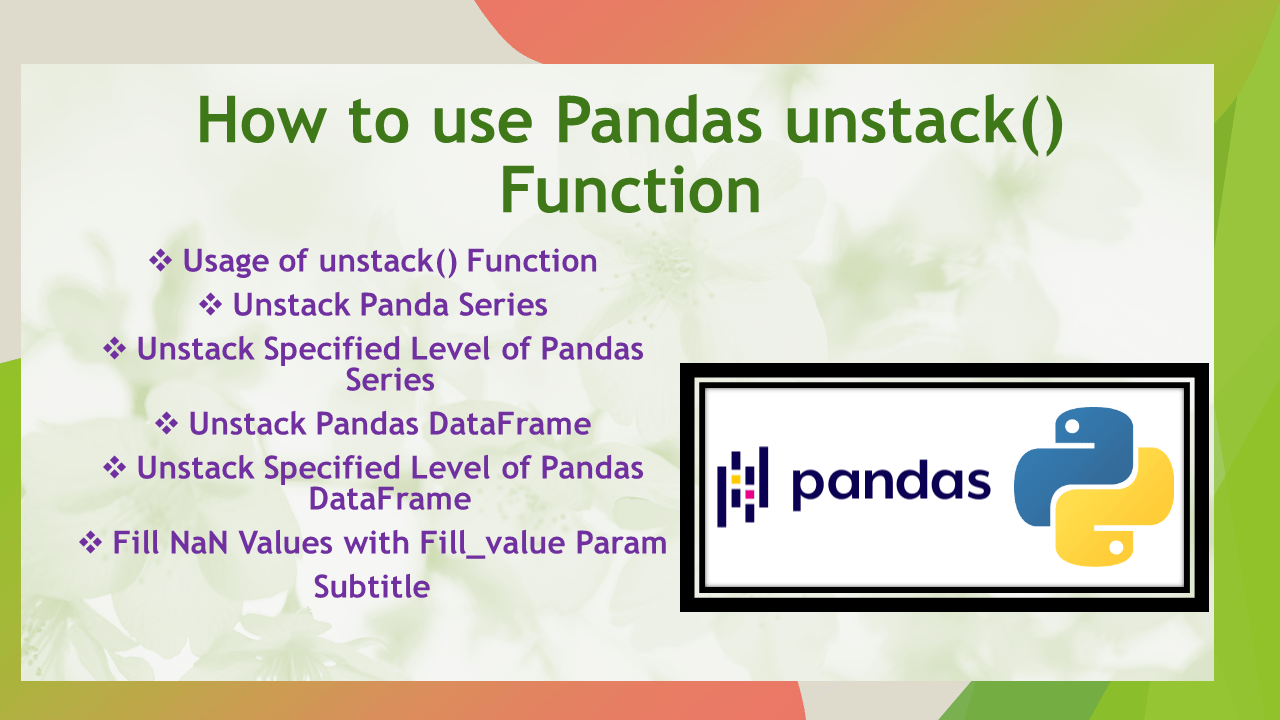# How to use Pandas unstack() Function

• Post author:
• Post category:Pandas

`Pandas.DataFrame.unstack()` is used to reshape the given Pandas DataFrame by transposing specified row level to column level. By default, it transposes the innermost row level into a column level. This is one of the technique for reshaping the DataFrame. When we want to analyze or reshape the data, Pandas provides in-built functions. Among those functions stack() and unstack() functions are the most popular functions for transposing row level to column and vice versa.

In this article, I will explain Pandas `unstack()` function and using its syntax and parameters how we can transpose the single/multi-level row to column level with examples.

## 1. Quick Examples of unstack() Function

If you are in a hurry, below are some quick examples unstack() function.

``````
# Below are the quick examples

# Example 1: Unstack default level(-1) of pandas Series
print("Unstacked DataFrame :\n", ser.unstack())

# Example 2: Unstack specified level
print("Unstacked DataFrame :\n", ser.unstack(level = 0))

# Example 3: Unstack default level(-1) of pandas DataFrame
print("Unstacked DataFrame :\n", df.unstack())

# Example 4: Unstack specified level
print("Unstacked DataFrame :\n", df.unstack(level = 0))

# Example 5: Fill NaN value set fill_value
print("Unstacked DataFrame :\n", df.unstack(level = 0, fill_value = '-'))
``````

## 2. Syntax of Pandas unstack()

Following is the syntax of unstack() function.

``````
# Syntax of Pandas unstack()
DataFrame.unstack(level=- 1, fill_value=None)
``````

### 2.1 Parameters

Following are the parameters of unstack() function. It has two parameters.

• `level` `:` (int, str, list of int, and list of str)By default, it is set to -1 i.e. the last level can be unstacked. If we pass the specified level, it will unstack those levels.
• `fill_value :` ( int, str or dict) It replaces NaN values( which are produced from unstacking) with specified values.

### 2.2 Return Value

It returns an unstacked DataFrame.

## 3. Usage of unstack() Function

In Pandas `df.unstack()` function reshapes the given DataFrame by converting the row index to a column label. It returns a transposed DataFrame. This function is reverse to stack() function where stacking is done from column level to row level.

Let’s create Pandas Series with a multi-level index and apply the Pandas `unstack()` function, it will return the unstacked Pandas DataFrame. Set the index of multiple level using pandas.MultiIndex.from_tuples().

``````
# Create multi index Pandas Series
import pandas as pd
index = pd.MultiIndex.from_tuples([
('Seattle', 'Date'),
('Seattle', 'Temp'),
('Sanfrancesco', 'Date'),
('Sanfrancesco', 'Temp')
])
ser = pd.Series(["30-12-2010", 40.7, "31-12-2010",  40.5], index=index)
print(ser)
``````

Yields below output.

### 3.1 Unstack Panda Series

Apply `unstack()` function on a multi-indexed Pandas Series, by default it unstacked innermost row level to column level. It returns the unstacked DataFrame where the column labels are the innermost row indexes of the original Series.

``````
# Unstack default level(-1) of pandas Series
print("Unstacked DataFrame :\n", ser.unstack())
``````

## 4. Unstack Specified Level of Pandas Series

As we know from the above, by default(level = -1) it will unstack the innermost row level onto a column level. When we want to unstack a specified level, we can set `level` param with a specified level or list of levels. It will unstack specified row level to column level. For example,

``````
# Unstack specified level
print("Unstacked DataFrame :\n", ser.unstack(level = 0))

# Output:
# Unstacked DataFrame :
#       Sanfrancesco     Seattle
# Date   31-12-2010  30-12-2010
# Temp         40.5        40.7
``````

## 5. Unstack Pandas DataFrame

Apply `unstack()` function on a multi-indexed Pandas DataFrame. It returns the unstacked DataFrame where the column labels are the innermost row indexes of original DataFrame.

Let’s create Pandas DataFrame with multi-level index,

``````
# Unstack default level(-1) of pandas DataFrame
multi_index = pd.MultiIndex.from_tuples([("Index1","Seattle"),
("Index1","Sanfrancesco"),
("Index2","Newyork"),
("Index2","Washington")])
df = pd.DataFrame({"Date":["30-12-2010",
"30-12-2010",
"30-12-2010",
"30-12-2010"],"Temp":[40.2, 40.5, 41.4, 42.1]}, index=multi_index)

print(df)
print("Unstacked DataFrame :\n", df.unstack())

# Output:
#                           Date  Temp
# Index1 Seattle       30-12-2010  40.2
#       Sanfrancesco  30-12-2010  40.5
# Index2 Newyork       30-12-2010  41.4
#       Washington     30-12-2010  42.1
#

# Unstacked DataFrame :
#               Date                           ...         Temp
#           Newyork Sanfrancesco     Seattle  ... Sanfrancesco Seattle Washington
# Index1         NaN   30-12-2010  30-12-2010  ...         40.5    40.2       NaN
# Index2  30-12-2010          NaN         NaN  ...          NaN     NaN      42.1
#
# [2 rows x 8 columns]
``````

## 6. Unstack Specified Level of Pandas DataFrame

The below example will unstack the specified row level to the column level. For example,

``````
# Unstack specified level
print("Unstacked DataFrame :\n", df.unstack(level = 0))

# Output:
#                      Date               Temp
#                  Index1      Index2 Index1 Index2
# NewYork              NaN  30-12-2010    NaN   41.4
# Sanfrancesco  30-12-2010         NaN   40.5    NaN
# Seattle       30-12-2010         NaN   40.2    NaN
# Washington            NaN  30-12-2010    NaN   42.1
``````

## 7. Fill NaN Values with Fill_value Param

Use `fill_value` param with specified value into unstack() function to replace NaN value with a specific value.

``````
# Fill NaN value set fill_value
print("Unstacked DataFrame :\n", df.unstack(level = 0, fill_value = '-'))

# Output:
# Unstacked DataFrame :
#                      Date               Temp
#                  Index1      Index2 Index1 Index2
# Newyork                -  30-12-2010      -   41.4
# Sanfrancesco  30-12-2010           -   40.5      -
# Seattle       30-12-2010           -   40.2      -
# Washington              -  30-12-2010      -   42.1
``````

## 8. Conclusion

In this article, I have explained the Pandas `unstack()` function and using its syntax and parameters how we can transpose row level to column level in a Series/DataFrame with examples.

Happy learning!!

## References

### Naveen

I am a Data Engineer with 20+ years of experience in transforming data into actionable insights. Over the years, I have honed my expertise in designing, implementing, and maintaining data pipelines with frameworks like Apache Spark, PySpark, Pandas, R, Hive and Machine Learning. My journey in the field of data engineering has been a continuous learning, innovation, and a strong commitment to data integrity. I have started this SparkByExamples.com to share my experiences with the data as I come across. You can learn more about me at LinkedInPandas unstack() function